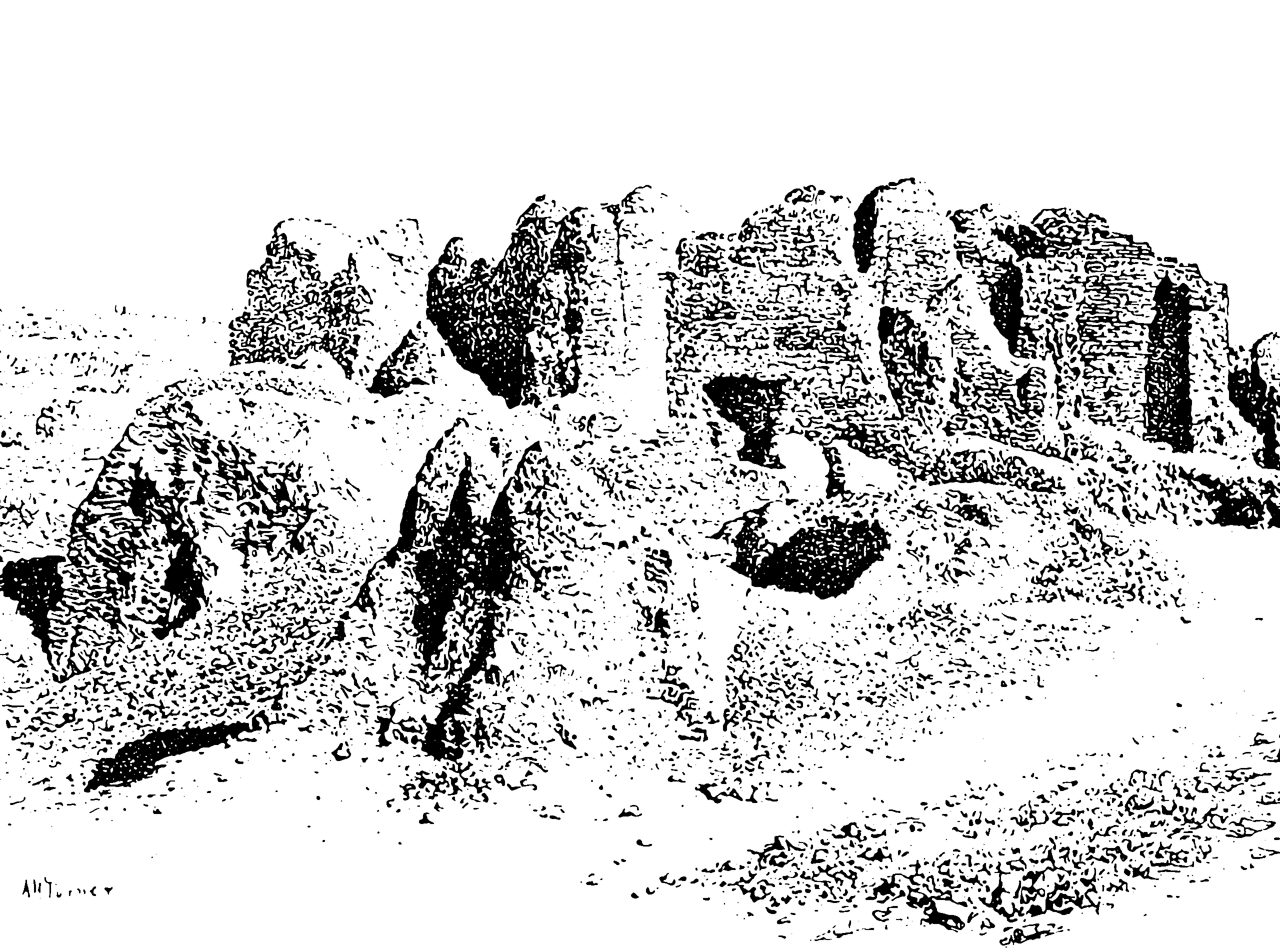# Measure-valued random variates

## Including completely random measures and many generalizationsOften I need to have a nonparametric representation for a measure over some non-finite index set. We might want to represent a probability, or mass, or a rate. I might want this representation to be something flexible and low-assumption, like a Gaussian process. If I want a nonparametric representation of functions this is not hard; I can simply use a Gaussian process. What can I use for measures? If I am working directly with random distributions of (e.g. probability) mass then I might want conservation of mass, for example.

Processes are that naturally represent mass and measure are a whole field in themselves. Giving a taxonomy is not easy, but the same ingredients and tools tend to recur; Here is a list of pieces that we can plug together to create a random measure.

## Completely random measures

See Kingman (1967) for the OG introduction. Foti et al. (2013) summarises:

A completely random measure (CRM) is a distribution over measures on some measurable space $$\left(\Theta, \mathcal{F}_{\Theta}\right)$$, such that the masses $$\Gamma\left(A_{1}\right), \Gamma\left(A_{2}\right), \ldots$$ assigned to disjoint subsets $$A_{1}, A_{2}, \cdots \in \mathcal{F}_{\Theta}$$ by a random measure $$\Gamma$$ are independent. The class of completely random measures contains important distributions such as the Beta process, the Gamma process, the Poisson process and the stable subordinator.

AFAICT any subordinator will in do, i.e. any a.s. non-decreasing Lévy process.

TBC

## Dirichlet processes

Random locations plus random weights gives us a Dirichlet process. Breaking sticks, or estimation of probability distributions using the Dirichlet process. I should work out how to sample from the posterior of these. Presumably the Gibbs sampler from Ishwaran and James (2001) is the main trick.

## Random coefficient polynomials

As seen in random spectral measures. TBC

## Beta process

As seen, apparently, in survival analysis .

## Other

Various transforms of Gaussian processes seem popular, e.g. squared or exponentiated. These always seem too messy to me.

## Incoming

### No comments yet. Why not leave one?

GitHub-flavored Markdown & a sane subset of HTML is supported.